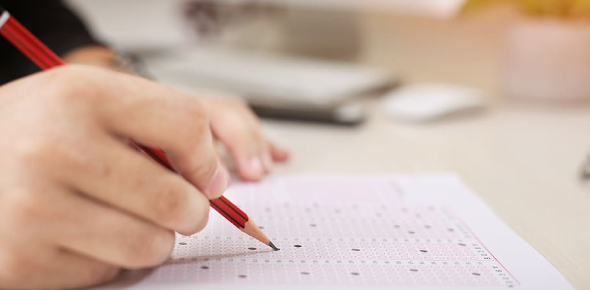# MCQ Set 1 - Transistor Fundamentals

31 Questions | Total Attempts: 1699Settings.

• 1.
The current gain of a transistor is defined as the ratio of the collector current to the
• A.

Base current

• B.

Emitter current

• C.

Supply current

• D.

Collector current

• 2.
The graph of current gain versus collector current indicates that the current gain
• A.

Is constant

• B.

Varies slightly

• C.

Varies significantly

• D.

Equals the collector current divided by the base current

• 3.
When the collector current increases, what does the current gain do?
• A.

Decreases

• B.

Stays the same

• C.

Increases

• D.

Any of the above

• 4.
As the temperature increases, the current gain
• A.

Decreases

• B.

Remains the same

• C.

Increases

• D.

Can be any of the above

• 5.
When the base resistor increases, the collector voltage will probably
• A.

Decrease

• B.

Stay the same

• C.

Increase

• D.

Do all of the above

• 6.
If the base resistor is very small, the transistor will operate in the
• A.

Cutoff region

• B.

Active region

• C.

Saturation region

• D.

Do all of the above

• 7.
Ignoring the bulk resistance of the collector diode, the collector-emitter saturation voltage is
• A.

0V

• B.

A few tenths of a volt

• C.

1V

• D.

Supply voltage

• 8.
Three different Q points are shown on a load line. The upper Q point represents the
• A.

Minimum current gain

• B.

Intermediate current gain

• C.

Maximum current gain

• D.

Cutoff point

• 9.
If a transistor operates at the middle of the load line, a decrease in the base resistance will move the Q point
• A.

Down

• B.

Up

• C.

Nowhere

• D.

• 10.
If the base supply voltage increase, the Q point moves
• A.

Down

• B.

Up

• C.

Nowhere

• D.

• 11.
Suppose the base resistor is open. The Q point will be
• A.

In the middle of the load line

• B.

At the upper end of the load line

• C.

At the lower end of the load line

• D.

• 12.
If the base supply voltage is disconnected, the collector-emitter voltage will equal
• A.

0V

• B.

6V

• C.

10.5V

• D.

Collector supply voltage

• 13.
If the base resistor has zero resistance, the transistor will probably be
• A.

Saturated

• B.

In cutoff

• C.

Destroyed

• D.

None of the above

• 14.
If the collector resistor opens in a base-biased circuit, the load line will become
• A.

Horizontal

• B.

Vertical

• C.

Useless

• D.

Flat

• 15.
The collector current is 1.5mA. If the current gain is 50, the base current is
• A.

3uA

• B.

30uA

• C.

150uA

• D.

3mA

• 16.
The base current is 50uA. If the current gain is 100, the collector current is closest in value to
• A.

50uA

• B.

500uA

• C.

2mA

• D.

5mA

• 17.
When the Q point moves along the load line, VCE decreases when the collector current
• A.

Decreases

• B.

Stays the same

• C.

Increases

• D.

Does none of the above

• 18.
When there is no base current in a transistor switch, the output voltage from the transistor is
• A.

Low

• B.

High

• C.

Unchanged

• D.

Unknown

• 19.
A circuit with a fixed emitter current is called
• A.

Base bias

• B.

Emitter bias

• C.

Transistor bias

• D.

Two-supply bias

• 20.
The first step in analyzing emitter-based circuits is to find the
• A.

Base current

• B.

Emitter current

• C.

Supply current

• D.

Collector current

• 21.
If the current gain is unknown in an emitter-biased circuit, you cannot calculate the
• A.

Emitter voltage

• B.

Emitter current

• C.

Collector current

• D.

Base current

• 22.
If the emitter resistor is open, the collector voltage is
• A.

Low

• B.

High

• C.

Unchanged

• D.

Unknown

• 23.
If the collector resistor is open, the collector voltage is
• A.

Low

• B.

High

• C.

Unchanged

• D.

Unknown

• 24.
When the current gain increases from 50 to 300 in an emitter-biased circuit, the collector current
• A.

Remains almost the same

• B.

Decreases by a factor of 6

• C.

Increases by a factor of 6

• D.

Is zero

• 25.
If the emitter resistance increases, the collector voltage
• A.

Decreases

• B.

Stays the same

• C.

Increases

• D.

Breaks down the transistor

Related TopicsBack to top